# Iterated monodromy group

Jump to: navigation, search

## Definition

### Motivation

The notion of iterated monodromy group is defined in the context of a covering map from a subspace of a space to the whole space (the covering map obviously differs from the inclusion of the subspace). The key word here is iterated -- the fact that the cover itself can also be identified as a subset of the space allows us to consider iterating the function used for the covering map.

### Full definition

Suppose$X$ is a path-connected locally path-connected topological space,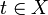$t \in X$,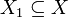$X_1 \subseteq X$, and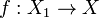$f:X_1 \to X$ is a covering map. We define the iterated monodromy group of$f$, denoted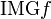$\operatorname{IMG} f$, as follows: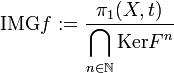$\operatorname{IMG} f := \frac{\pi_1(X,t)}\bigcap_{n \in \mathbb{N}} \operatorname{Ker} F^n$

where:

•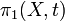$\pi_1(X,t)$ is the fundamental group of$X$ at basepoint$t$.
•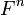$F^n$ represents the homomorphism from$\pi_1(X,t)$ to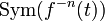$\operatorname{Sym}(f^{-n}(t))$ viewed in the context of the covering map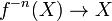$f^{-n}(X) \to X$. Note that in the case$n = 1$, we get the usual monodromy action for$F$ and the quotient by the kernel is the usual monodromy group.

In other words, the iterated monodromy group stores the fundamental group modulo those loops that act trivially on all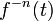$f^{-n}(t)$ sets.